# Array Creation ENGR 1181 MATLAB 2 Array Creation

• Slides: 31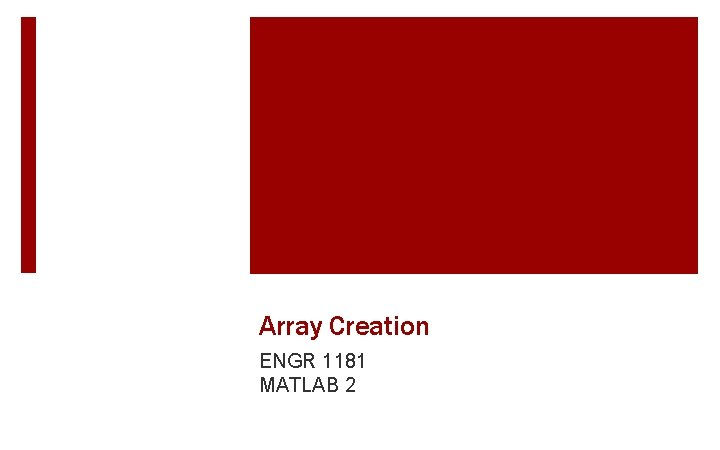Array Creation ENGR 1181 MATLAB 2Array Creation In The Real World Civil engineers store seismic data in arrays to analyze plate tectonics as well as fault patterns. These sets of data are critical to structural design in areas like California where earthquakes are prevalent and dangerous in number of occurrences and magnitude.Today’s Learning Objectives § After today’s class, students will be able to: • Demonstrate proper convention for assigning arrays to a variable (e. g. , space, semi-colons, colon operators, linspace function). • Apply the transpose operator correctly.What is a vector? A vector is an ordered list of several numbers and is a one-dimensional array § Row Vectors are horizontal: [1 2 3 4] § Column Vectors are vertical: [1 2 3 4]Examples of Vectors We could make a vector that consists of even numbers from 2 to 10: [2 4 6 8 10] We could make a vector contains average temperature data for four months: [28 33 42 51]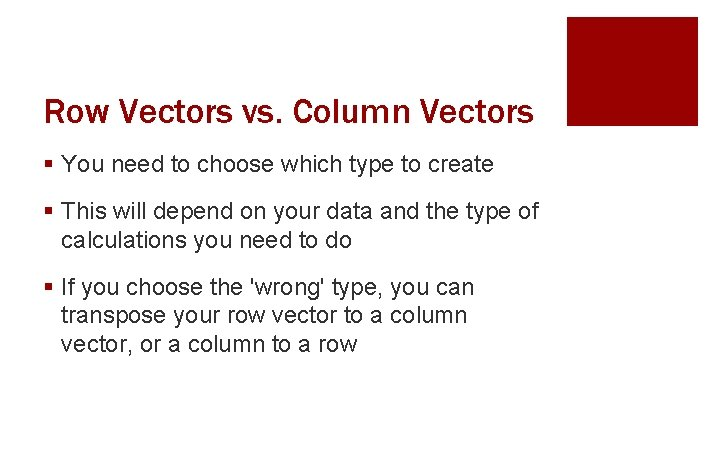Row Vectors vs. Column Vectors § You need to choose which type to create § This will depend on your data and the type of calculations you need to do § If you choose the 'wrong' type, you can transpose your row vector to a column vector, or a column to a row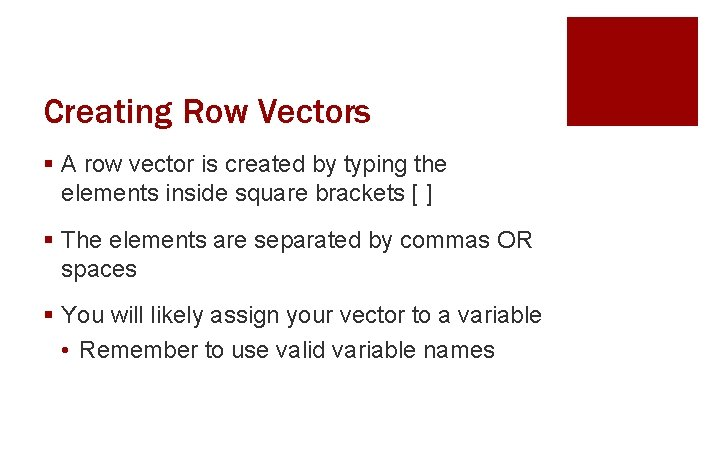Creating Row Vectors § A row vector is created by typing the elements inside square brackets [ ] § The elements are separated by commas OR spaces § You will likely assign your vector to a variable • Remember to use valid variable names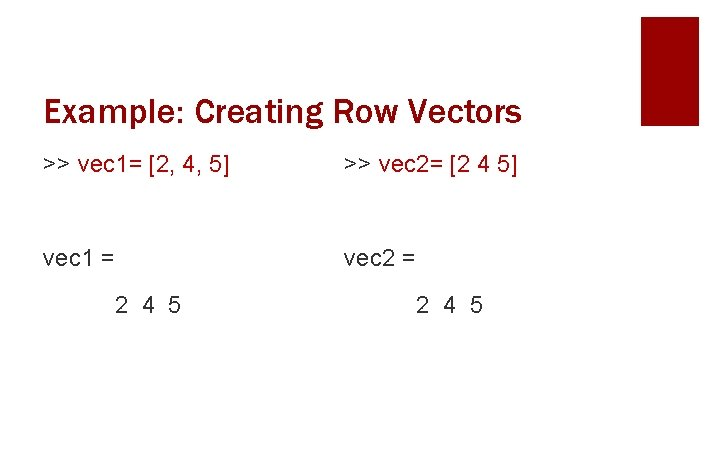Example: Creating Row Vectors >> vec 1= [2, 4, 5] >> vec 2= [2 4 5] vec 1 = vec 2 = 2 4 5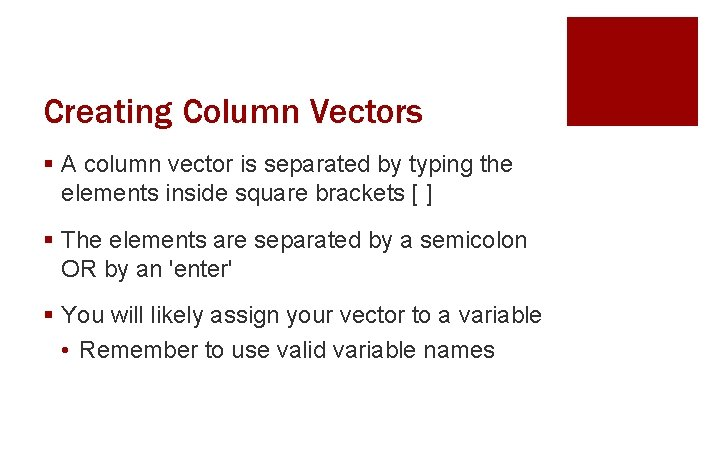Creating Column Vectors § A column vector is separated by typing the elements inside square brackets [ ] § The elements are separated by a semicolon OR by an 'enter' § You will likely assign your vector to a variable • Remember to use valid variable names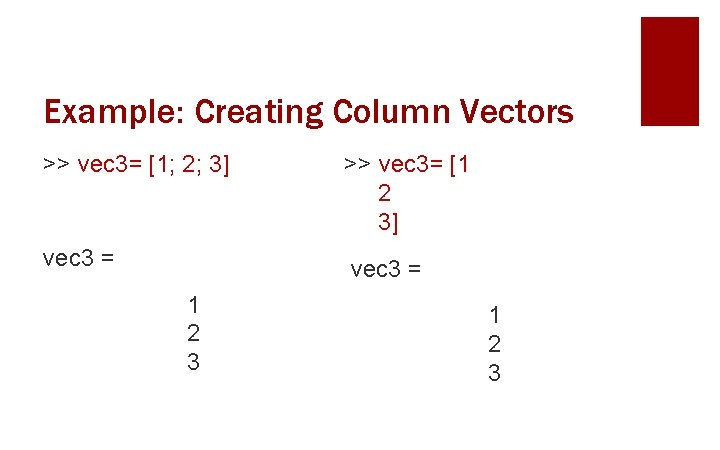Example: Creating Column Vectors >> vec 3= [1; 2; 3] >> vec 3= [1 2 3] vec 3 = 1 2 3Transposing Vectors § We can change a row vector into a column, or a column into a row § This is called transposing § We use a single quote to do this: ′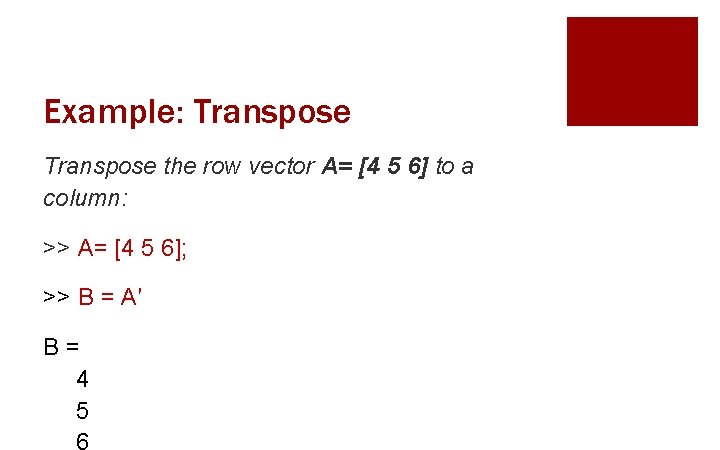Example: Transpose the row vector A= [4 5 6] to a column: >> A= [4 5 6]; >> B = A′ B= 4 5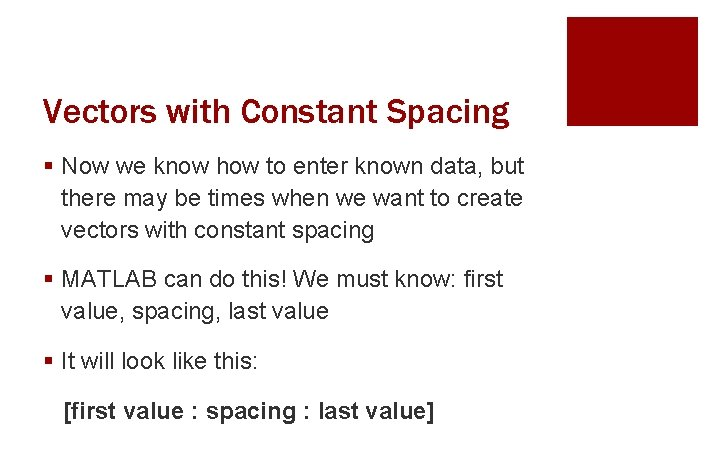Vectors with Constant Spacing § Now we know how to enter known data, but there may be times when we want to create vectors with constant spacing § MATLAB can do this! We must know: first value, spacing, last value § It will look like this: [first value : spacing : last value]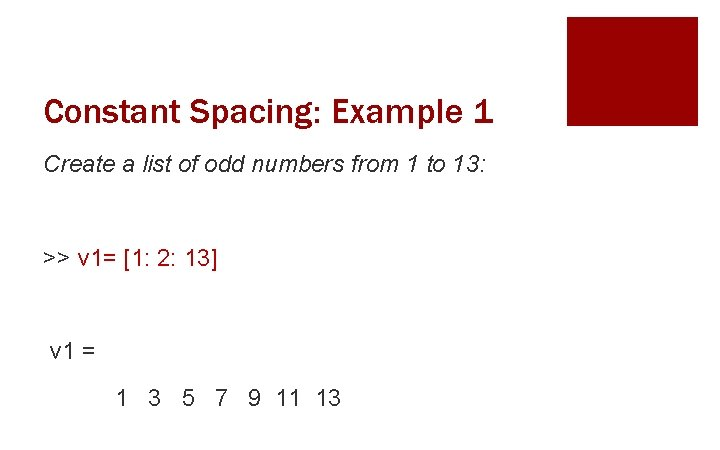Constant Spacing: Example 1 Create a list of odd numbers from 1 to 13: >> v 1= [1: 2: 13] v 1 = 1 3 5 7 9 11 13Constant Spacing: Example 2 Create a vector called v 3 with numbers divisible by 7 from -63 to -28. >> v 3 = [ -63 : 7 : -28] v 3 = -63 -56 -49 -42 -35 -28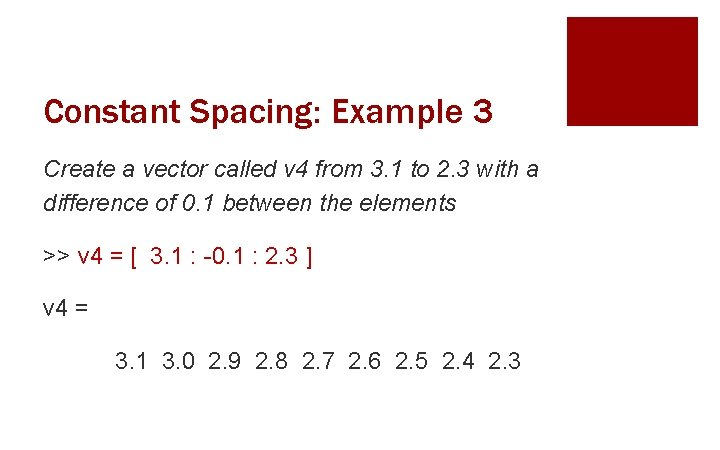Constant Spacing: Example 3 Create a vector called v 4 from 3. 1 to 2. 3 with a difference of 0. 1 between the elements >> v 4 = [ 3. 1 : -0. 1 : 2. 3 ] v 4 = 3. 1 3. 0 2. 9 2. 8 2. 7 2. 6 2. 5 2. 4 2. 3Constant Spacing Default Value If we do not specify a spacing value, MATLAB will default to a spacing value of 1: >> v 2= [3: 7] v 2 = 34567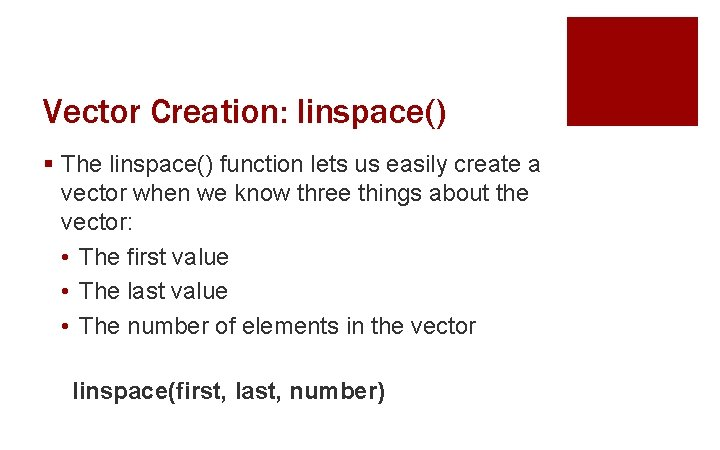Vector Creation: linspace() § The linspace() function lets us easily create a vector when we know three things about the vector: • The first value • The last value • The number of elements in the vector linspace(first, last, number)Example: Linspace Function Create a vector v 5 with 6 elements, starting at 2. 5 and ending at 3. 5: >> v 5 = linspace(2. 5, 3. 5, 6) v 5 = 2. 50 2. 70 2. 90 3. 10 3. 30 3. 50Example: Linspace Function Create a vector called v 6 with 10 elements, starting at 99 and ending at 33: >> v 6 = linspace(99, 33, 10) v 6 = 99. 0 91. 7 84. 3 77. 0 69. 7 62. 3 55. 0 47. 6 40. 3 33. 0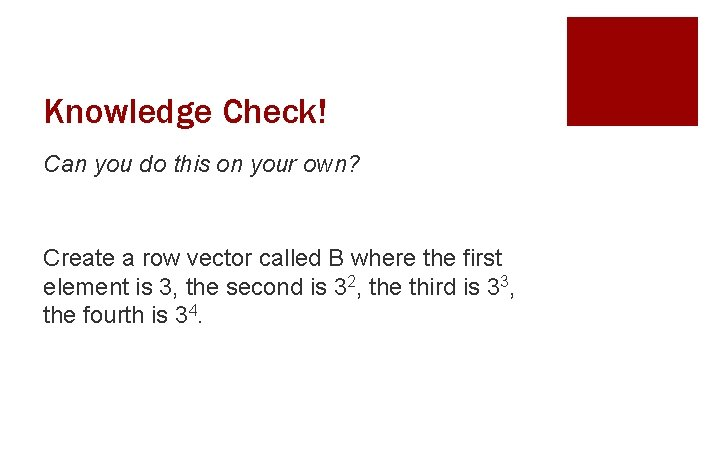Knowledge Check! Can you do this on your own? Create a row vector called B where the first element is 3, the second is 32, the third is 33, the fourth is 34.Knowledge Check! Create a row vector called B where the first element is 3, the second is 32, the third is 33, the fourth is 34. >> B = [3 3^2 3^3 3^4] B= 3 9 27 81Knowledge Check! … Is there any other way we could have created B? >> x=3; >> B= [x x^2 x^3 x^4] B= 3 9 27 81Matrices: 2 -D Arrays A matrix is a two-dimensional representation of a group of numbers containing rows and columns You can think of it as many row vectors stacked on top of one another 2 4 6 8 1 2 3 4Matrix Example We have attendance data for number of absences of three students over 4 months. We can create this as a matrix: Jan Attendance Feb 10 10 9 7 7 10 Mar Apr 9 6 7 8 9 6 Student A Student B Student CMatrix Size § The size of a matrix is described by the number of rows and columns it contains. § A (m x n) matrix has m rows and n columns. • Referred to as an “m by n” matrix • Attendance is a 3 by 4 matrix Attendance 9 7 10 10 9 7 6 9 10 7 6 8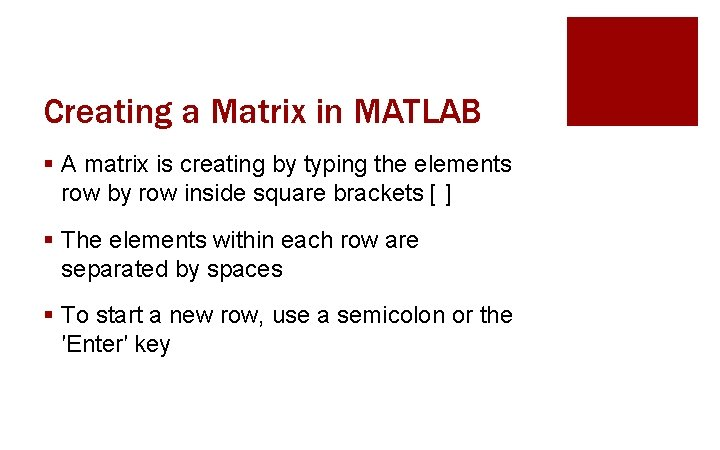Creating a Matrix in MATLAB § A matrix is creating by typing the elements row by row inside square brackets [ ] § The elements within each row are separated by spaces § To start a new row, use a semicolon or the ′Enter′ key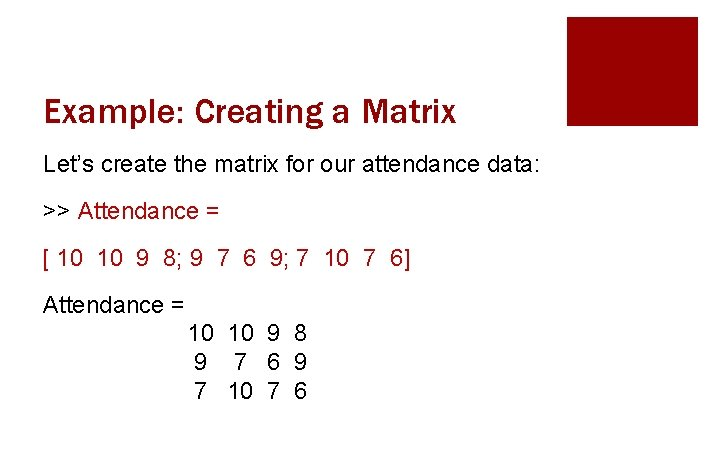Example: Creating a Matrix Let’s create the matrix for our attendance data: >> Attendance = [ 10 10 9 8; 9 7 6 9; 7 10 7 6] Attendance = 10 10 9 8 9 7 6 9 7 10 7 6Important Takeaways § Row vectors are horizontal with only 1 row § Elements are entered separated by commas or spaces § Column vectors are vertical with only 1 column, matrices have multiple columns and rows, rows are entered separated by a semicolon or ′Enter′ key § A matrix is a two-dimensional array. Its size is described as (m x n), or “rows by columns”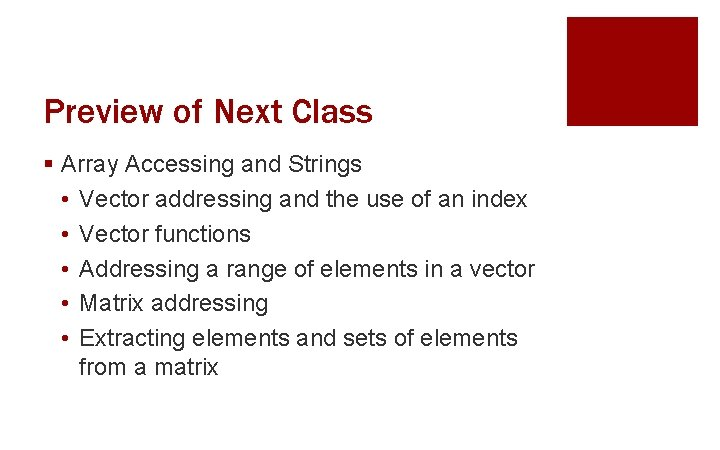Preview of Next Class § Array Accessing and Strings • Vector addressing and the use of an index • Vector functions • Addressing a range of elements in a vector • Matrix addressing • Extracting elements and sets of elements from a matrix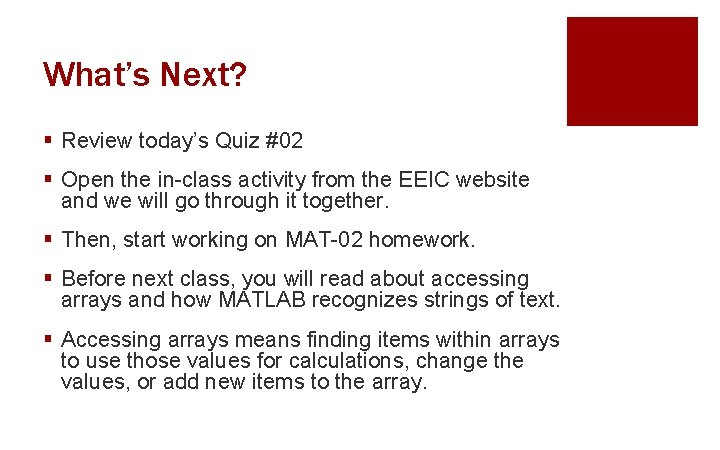What’s Next? § Review today’s Quiz #02 § Open the in-class activity from the EEIC website and we will go through it together. § Then, start working on MAT-02 homework. § Before next class, you will read about accessing arrays and how MATLAB recognizes strings of text. § Accessing arrays means finding items within arrays to use those values for calculations, change the values, or add new items to the array.